# I'm struggling with an Arithmetic Geometrical question.

## Homework Statement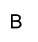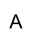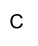The angles in triangle ABC form an increasing arithmetic sequence.

The ratio of angles A:B:C can be written in the form 185:370:555 respectively.

You are told that the total area of the triangle is 9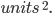Length BC is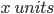Given the area of triangle ABC, work out the value of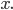Give your answer in the form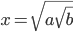whereand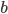are integers.

You must not make any assumptions

You must show all your working

## Homework Equations

[/B]
I've attempted to use ##1/2*ab*SinC## presumed it's a right angle.
Area of a triangle: ##1/2*bh##.
Pythagorean Law.

## The Attempt at a Solution

I've attempted to firstly figure out the angles of the triangle. I know that they form an increasing arithmetic sequence. So I've used a multiplier of 6/37 which I obtained by:
Let multiplier = ##y##
##y(185+370+555) = 180##
##1110y=180##
##y = 6/37##
On the diagram the angles aren't exactly labelled, so on the bottom left its angle A, bottom right is Angle C and top right is Angle B.
Angle A: ##6/37*185 = 30##
Angle B: ##6/37*370 = 60##
Angle C: ##6/37*555 = 90##
##30, 60, 90## are an increasing sequence by ##+30## each time.

BC = ##x##
Using Trig, ##Sin(30) = Opp/Hyp## Hypotenuse (BA) is ##2x##.
Using Law of Pythagorean = ##a^2+b^2 = c^2##
##(2x)^2 - x^2 = AC##
##4x^2 - x^2 = AC##
##3x^2= AC##
##AC= \sqrt 3 x##
Not sure how to progress from here. Any guidance? Thank you.

[/B]

Ray Vickson
Science Advisor
Homework Helper
Dearly Missed

## Homework Statement

View attachment 222981

View attachment 222982

View attachment 222983View attachment 222984

The angles in triangle ABC form an increasing arithmetic sequence.

The ratio of angles A:B:C can be written in the form 185:370:555 respectively.

You are told that the total area of the triangle is 9View attachment 222985

Length BC is View attachment 222986

Given the area of triangle ABC, work out the value of View attachment 222987

Give your answer in the form View attachment 222988 where View attachment 222989and View attachment 222990are integers.

You must not make any assumptions

You must show all your working

## Homework Equations

[/B]
I've attempted to use ##1/2*ab*SinC## presumed it's a right angle.
Area of a triangle: ##1/2*bh##.
Pythagorean Law.

## The Attempt at a Solution

I've attempted to firstly figure out the angles of the triangle. I know that they form an increasing arithmetic sequence. So I've used a multiplier of 6/37 which I obtained by:
Let multiplier = ##y##
##y(185+370+555) = 180##
##1110y=180##
##y = 6/37##
On the diagram the angles aren't exactly labelled, so on the bottom left its angle A, bottom right is Angle C and top right is Angle B.
Angle A: ##6/37*185 = 30##
Angle B: ##6/37*370 = 60##
Angle C: ##6/37*555 = 90##
##30, 60, 90## are an increasing sequence by ##+30## each time.

BC = ##x##
Using Trig, ##Sin(30) = Opp/Hyp## Hypotenuse (BA) is ##2x##.
Using Law of Pythagorean = ##a^2+b^2 = c^2##
##(2x)^2 - x^2 = AC##
##4x^2 - x^2 = AC##
##3x^2= AC##
##AC= \sqrt 3 x##
Not sure how to progress from here. Any guidance? Thank you.
[/B]

You were told the area of the triangle; use that information to complete the calculations.

BTW: in LaTeX the trig functions should be written with a "\" in front; that gives you ##\sin 30## or ##\sin(30)## instead of the ugly and hard-to-read ##sin(30)## or ##sin 30##. (Just right-click on a formula to see its TeX/LateX inputs).

Charles Link
Homework Helper
Gold Member
2020 Award
Look at the ratios 185:370:555 . Can you write those in lowest terms?

I've reached up to ##b*h/2 = 9##.
Will it equal ##\sqrt 3 x*x \2 = 9##?

•Charles Link
Charles Link
Homework Helper
Gold Member
2020 Award
I've attempted that now, don't they all cancel out to zero?
What do you get when you divide each number by 185?

What do you get when you divide each number by 185?
##1:2:3##? I'm trying to find the value of ##x## though, what difference is it by looking at the ratios? I've found out each angle from it..

•Charles Link
Charles Link
Homework Helper
Gold Member
2020 Award
##1:2:3##?
You got the same answer, the hard way, that the triangle is a ##30^o##, ##60^o##, ##90^o ##. And the result of your latest calculation looks correct to me. A little simplifying into the necessary form should get you there.

•Physiona
You got the same answer, the hard way, that the triangle is a 30, 60, 90. And the result of your latest calculation looks correct to me. A little simplifying into the necessary form should get you there.
So is post #4 correct so far? If so, how do I simplify the surd form of ##\sqrt 3x*x=9##? The coefficients aren't similar so I do I obtain ##x=..##? Because I thought it'd be ##\sqrt 3 x^2 = 9## but I don't think it's mathematically correct.

Charles Link
Homework Helper
Gold Member
2020 Award
Do you know that ## \sqrt{3}=3^{1/2} ##?

•Physiona
nomadreid
Gold Member
You've got the angles OK, & the sides are OK. Now, you can get the area simply by thinking of your triangle as half of a rectangle with sides AC & BC ((flip and rotate your triangle and put the two hypothenuses together), so your formula simply becomes AC*BC/2=(√3x)x/2 =9. Solve for x. The necessary form comes out directly. (Note: 9/√3 = 3(√3)2/√3 is one possible step.)

•Physiona
Charles Link
Homework Helper
Gold Member
2020 Award
So is post #4 correct so far? If so, how do I simplify the surd form of ##\sqrt 3x*x=9##? The coefficients aren't similar so I do I obtain ##x=..##? Because I thought it'd be ##\sqrt 3 x^2 = 9## but I don't think it's mathematically correct.
It's ## (\frac{1}{2})(\sqrt{3}) x^2=9 ##. You had it correct in post 4.

•Physiona
nomadreid
Gold Member
Note that multiplication is associative: (√3x)x =√3(x*x)

Note that I edited my above post for the forgotten 1/2 (thanks, Charles Link)

You've got the angles OK, & the sides are OK. Now, you can get the area simply by thinking of your triangle as half of a rectangle with sides AC & BC ((flip and rotate your triangle and put the two hypothenuses together), so your formula simply becomes AC*BC=(√3x)x =9. Solve for x. The necessary form comes out directly. (Note: 9/√3 = 3(√3)2/√3 is one possible step.)
So can it equal ##(\sqrt 3 x)x = 9##?
Then it equals ## 9/\sqrt 3 x##
( I don't get the ##3(\sqrt 3)^2/ \sqrt 3##)

Charles Link
Homework Helper
Gold Member
2020 Award
Suggest writing ## 9=3^2 ## and computing ## \frac{3^2}{3^{1/2}} ## as ## 3^u ## for some fraction ## u ##. Also notice the form they want: ## x=\sqrt{a \sqrt{b}}=a^{1/2}b^{1/4} ##.

•Physiona
It's ## (\frac{1}{2})(\sqrt{3}) x^2=9 ##. You had it correct in post 4.
Would it equal from there ##(\sqrt 3)*x^2 = 18##?
The thing is one length is ##\sqrt 3 x## multiplying it by ##x## will equal ##(\sqrt 3) x^2##?

Charles Link
Homework Helper
Gold Member
2020 Award
Get all the numbers on the right side and solve for ## x ## by taking a square root of both sides, which is the same thing as an exponent of ## (1/2) ## on both sides: i.e. Write it as ## x^2=A ##, and then ## (x^2)^{1/2}=x=A^{1/2} ##.

•Physiona
Get all the numbers on the right side and solve for ## x ## by taking a square root of both sides, which is the same thing as an exponent of ## (1/2) ## on both sides: i.e. Write it as ## x^2=A ##, and then ## (x^2)^{1/2}=x=A^{1/2} ##.
##1/2* (\sqrt 3 x)*x^2 = 9##
I can't do it from here. With linking to your guidance, which part do I begin from? The ##(\sqrt 3 x)* x^2## is frustrating. Will it equal ##(\sqrt 3 x^2)##?

Charles Link
Homework Helper
Gold Member
2020 Award
##1/2* (\sqrt 3 x)*x^2 = 9##
I can't do it from here. With linking to your guidance, which part do I begin from? The ##(\sqrt 3 x)* x^2## is frustrating.
Let me get you to the next step:## \\ ## ## (\frac{1}{2})(\sqrt{3})x^2=9 ## ## \\ ## Putting the numbers to one side gives ## \\ ## ## x^2=2 (\frac{3^2}{3^{1/2}})=(2 )(3^{3/2} )##. ## \\ ## The next part is to take the square root of both sides. That will give ## 3^{3/4} ## on the second term on the right, and you need it in the form ## b^{1/4} ##. (see also post 14). How can you convert this? What is ## b ##?

•Physiona
Let me get you to the next step:## \\ ## ## (\frac{1}{2})(\sqrt{3})x^2=9 ## ## \\ ## Putting the numbers to one side gives ## \\ ## ## x^2=2 (\frac{3^2}{3^{1/2}})=(2 )(3^{3/2} )##. ## \\ ## The next part is to take the square root of both sides. That will give ## 3^{3/4} ## on the second term on the right, and you need it in the form ## b^{1/4} ##. (see also post 14). How can you convert this? What is ## b ##?
Right I've understood it up to ##x^2 = 2* 3^{3/2}##.
##x = (\sqrt 2* 3^{3/2})##

Charles Link
Homework Helper
Gold Member
2020 Award
Right I've understood it up to ##x^2 = 2* 3^{3/2}##.
##x = (\sqrt 2* 3^{3/2})##
## x=(\sqrt{2}) (3^{3/4}) =(2^{1/2})(3^{3/4} ) ##. Looking at post 14, how do we convert that second term, ## 3^{3/4} ## to get it to the form ## b^{1/4} ## ? ## \\ ## (I'm really not supposed to tell you the answer, but you should be able to figure it out).

•Physiona
## x=(\sqrt{2}) (3^{3/4}) =(2^{1/2})(3^{3/4} ) ##. Looking at post 14, how do we convert that second term, ## 3^{3/4} ## to get it to the form ## b^{1/4} ## ? ## \\ ## (I'm really not supposed to tell you the answer, but you should be able to figure it out).
Okay thank you, I'll attempt it and I'll get back to you on this forum.

## x=(\sqrt{2}) (3^{3/4}) =(2^{1/2})(3^{3/4} ) ##. Looking at post 14, how do we convert that second term, ## 3^{3/4} ## to get it to the form ## b^{1/4} ## ? ## \\ ## (I'm really not supposed to tell you the answer, but you should be able to figure it out).
So after I have converted it how will it be in the standard form they are requiring?

Charles Link
Homework Helper
Gold Member
2020 Award
So after I have converted it how will it be in the standard form they are requiring?
Post 14 shows you that. Basically, you should recognize already that ## a=2 ##. The ## b ## is the very last step that you need to convert. It seems you have very limited practice with exponents, so let me show you the result: ## 3^{3/4}=(3^3)^{1/4} ##. Do you see why? Note: ## (y^u)^v=y^{uv} ##. ## \\ ## All that remains is to calculate ## b=3^3 ##. What number is ## b ##?

Post 14 shows you that. Basically, you should recognize already that ## a=2 ##. The ## b ## is the very last step that you need to convert. It seems you have very limited practice with exponents, so let me show you the result: ## 3^{3/4}=(3^3)^{1/4} ##. Do you see why? ## (x^u)^v=x^{uv} ##. All that remains is to calculate ## b=3^3 ##. What number is ## b ##?
Yes I was going to result to that as being ## b= 27 ##. My teacher hasn't taught these immediate methods with exponents quite well, and I've forgotten to do them. Either way, thank you for the helpful guidance. Much appreciated.

•Charles Link